# Algebra 1 : How to find the degree of a polynomial

## Example Questions

← Previous 1 3 4 5 6 7 8

### Example Question #1 : How To Find The Degree Of A Polynomial

Give the degree of the polynomial.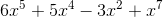Explanation:The degree of an individual term of a polynomial is the exponent of its variable; the exponents of the terms of this polynomial are, in order, 5, 4, 2, and 7.

The degree of the polynomial is the highest degree of any of the terms; in this case, it is 7.

### Example Question #2 : How To Find The Degree Of A Polynomial

What is the degree of the polynomial?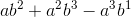Explanation:To find the degree of the polynomial, you first have to identify each term [term is for example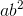], so to find the degree of each term you add the exponents.

EX: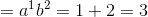- Degree of 3

Highest degree is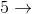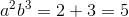### Example Question #1 : How To Find The Degree Of A Polynomial

What is the degree of the polynomial?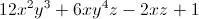Explanation:

To find the degree of the polynomial, add up the exponents of each term and select the highest sum.

12x2y3: 2 + 3 = 5

6xy4z: 1 + 4 + 1 = 6

2xz: 1 + 1 = 2

The degree is therefore 6.

### Example Question #1 : How To Find The Degree Of A Polynomial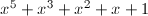What is the degree of the polynomial?Explanation:

The degree is the highest exponent value of the variables in the polynomial.

Here, the highest exponent is x5, so the degree is 5.

### Example Question #3 : How To Find The Degree Of A Polynomial

Which of the following depicts an equation in standard form?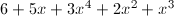None of the other answers are correct.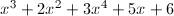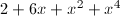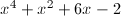Explanation:

A polynomial in standard form is written in descending order of the power. The highest power should be first, and the lowest power should be last.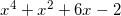The answer has the powers decreasing from four, to two, to one, to zero.

### Example Question #6 : How To Find The Degree Of A Polynomial

Simplify: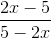-1

1

2x

None of the above

5

-1

Explanation:

The given expression can be re-written as: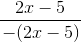Cancel (2x - 5):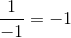### Example Question #1 : How To Find The Degree Of A Polynomial

Find the degree of the polynomial: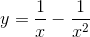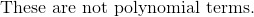Explanation:

The polynomial terms may only have variables raised to positive integer exponents.  No square roots, fraction powers, and variables in the denominator are allowed.### Example Question #1 : How To Find The Degree Of A Polynomial

What is the degree of the following polynomial?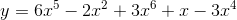Explanation:

The degree of the polynomial is the highest power in the polynomial.

The highest power given is the term: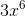Therefore, the degree of the polynomial is.

### Example Question #9 : How To Find The Degree Of A Polynomial

What is the degree of following polynomial?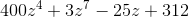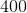Explanation:

The degree of a polynomial with a single variable (in our case,), simply find the largest exponent of that variable within the expression. The term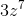showsbeing raised to the seventh power, and no otherin this expression is raised to anything larger than seven. Therefore, the degree of this expression is.

### Example Question #10 : How To Find The Degree Of A Polynomial

What is the degree of the following polynomial?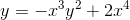Explanation:

The polynomial has more than one variable. The degree of the polynomial is the largest sum of the exponents of ALL variables in a term.

The first term is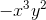.

The degree of this term is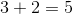The second term is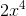.

The degree of this term is.

The degree of the polynomial is the largest of these two values, or.

← Previous 1 3 4 5 6 7 8